# Iterated Finite Automata

Posted to the NKS Forum November 17, 2003

I thought members of the Forum might find interesting something that arose from a discussion I had yesterday with Rostislav Grigorchuk (www.math.tamu.edu/~grigorch), a mathematician particularly known for his construction of groups of intermediate growth (see page 938 of the NKS book, and below).

Grigorchuk has studied connections between finite automata and group theory for more than 20 years. Key to what he has done is to look at transducer finite automata, and essentially to consider the effect of all possible iterations of them. I was curious whether there were systems based on finite automata whose explicit evolution could be studied like cellular automata—and talking to Grigorchuk I realized that iterated transducer finite automata give exactly this.

And in fact, such iterated finite automata seem like a rather nice systems, that I suspect are interesting not only for issues in pure mathematics, but also for other applications.

Any finite automaton can be represented by a network, in which each node is a state, and each edge represents a transition from one state to another (see e.g. page 957). These days the most common type of finite automaton considered are recognizer finite automata, in which there is a single symbol (say 0 or 1) on a given edge. The system then has the property that the sequence of symbols on each possible path through the network corresponds to a word in the regular language accepted by the finite automaton.

A transducer finite automaton, however, is set up to take in one sequence of symbols, and put out another. The way it works is to have both an input and an output symbol on each edge in the network. Transducer finite automata are sometimes known as Mealy machines, and particularly in the past, they were often used as models of electrical or mechanical machines that operate in a sequential way.

A convenient way to represent a transducer finite automaton is to specify each edge in its network by giving a rule of the form

{state1, input} -> {state2, output}.

Then an example of a 2-state, 2-symbol transducer finite automaton is:

fa = {{1, 1} -> {1, 0}, {1, 0} -> {2, 1}, {2, 1} -> {2, 1},
{2, 0} -> {1, 0}}

The following function takes a sequence list and applies a transducer finite automaton rule, starting in state s0:

FAApply[rule_, s0_, list_] :=
FoldList[{First[#1], #2} /. rule &, {s0}, list]

With input sequence {1, 0, 1, 1, 0, 0, 0}, and starting in state 1, this then yields output:

{{1}, {1, 0}, {2, 1}, {2, 1}, {2, 1}, {1, 0}, {2, 1}, {1, 0}}

If one looks only at the output sequence, the action of the transducer finite automaton is given by:

FAStep[rule_, s0_, list_] :=
Map[Last, Rest[FoldList[
{First[#1], #2}/. rule &, {s0}, list]]]

Now one can iterate this operation, as in:

FAEvolveList[rule_, s0_, init_, t_] :=
NestList[FAStep[rule, s0, #]&, init, t]

So consider for example:

Show[RasterGraphics[FAEvolveList[
fa, 1, Table[0, {100}], 60]]]

with

RasterGraphics[data_] :=
Graphics[Raster[1 - Reverse[data]],
AspectRatio -> Automatic]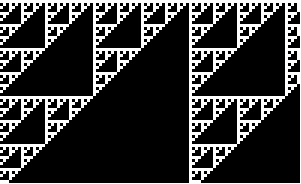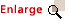The result—even from a blank tape—is a rule-90-like nested pattern. But the pattern is tipped on its side—and in fact is similar to the pattern from the rule 60 sequential cellular automaton on page 1034.

It is not surprising that there is some similarity between iterated finite automata and cellular automata. But an obvious difference is that while ordinary cellular automata fundamentally update cells in parallel, iterated finite automata do it sequentially. And this has the consequence that in an iterated finite automaton a particular cell can affect cells even arbitrarily far to its right. (It is like an infinite impulse response filter.)

Iterated finite automata seem potentially useful as models of various practical situations. But as a pure NKS question, one can ask what behavior occurs for iterated finite automata with all possible simple rules.

There are (k s)(k s) s-state, k-symbol transducer finite automata—or 256 2-state, 2-symbol ones. One can number finite automata in analogy to the numbering of Turing machines from page 888R. The rule corresponding to a finite automaton with number m is then:

ToFARule[m_Integer, {s_Integer, k_Integer}] :=
Flatten[MapIndexed[{1, -1}#2 + {0, k} ->
Mod[Quotient[#1, {k, 1}], {s, k}] + {1, 0} &,
Partition[IntegerDigits[m, s k, s k], k], {2}]]

The example given above is automaton 60 in this numbering scheme.

One can then make a picture of the behavior of all 2-state, 2-symbol iterated finite automata using:

Show[GraphicsArray[Partition[Table[
RasterGraphics[FAEvolveList[ToFARule[
m, {2, 2}], 1, Table[0, {100}], 60]], {m, 0, 255}], 8]]]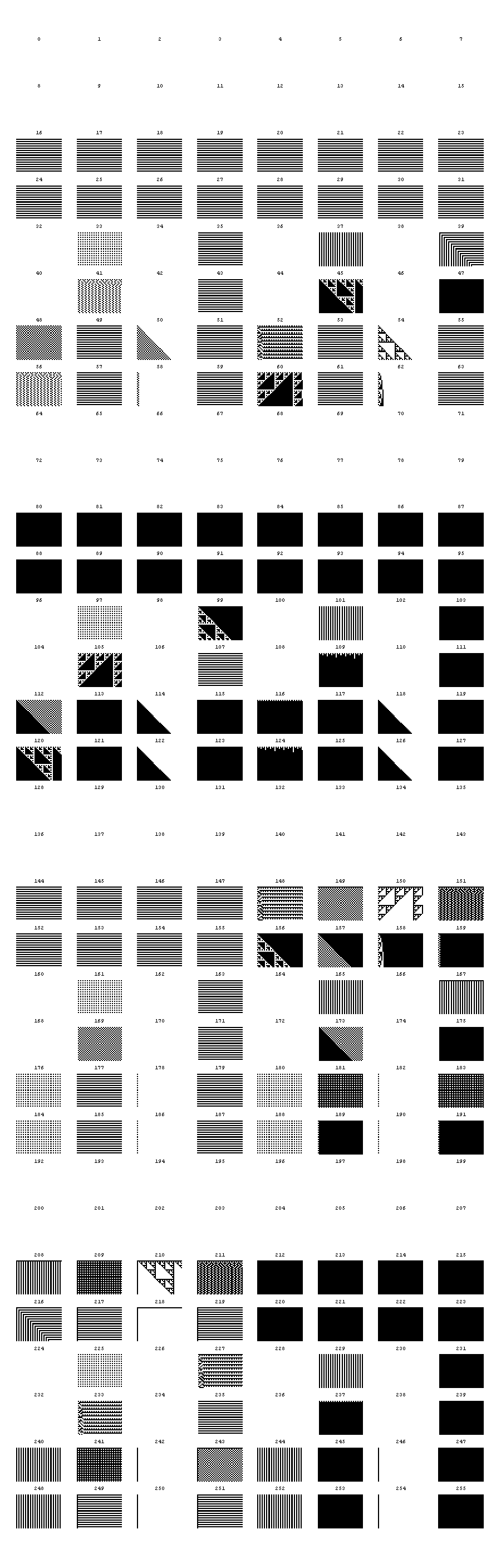The majority show very simple uniform or repetitive behavior. Automata 62 and 158 give IntegerDigits[t, 2] on step t, leading to logarithmic growth as on page 117. (The rule for 62 is {{1, 1} -> {1, 0}, {1, 0} -> {2, 1}, {2, 1} -> {2, 1}, {2, 0} -> {2, 0}}—exactly addition with carry.) Automata 227 and 233 give slightly modified versions of the same pattern. There are then 9 automata that yield nested patterns. Many show in effect infinite rate of information propagation. But for example automaton 54 shows exactly a rule 60 pattern.

What is needed to get more complicated behavior?

Let’s consider the case of 3 states. There are 66 = 46656 rules of this type. It is straightforward to search for ones that do not, for example, have purely repetitive behavior. A few thousand are left.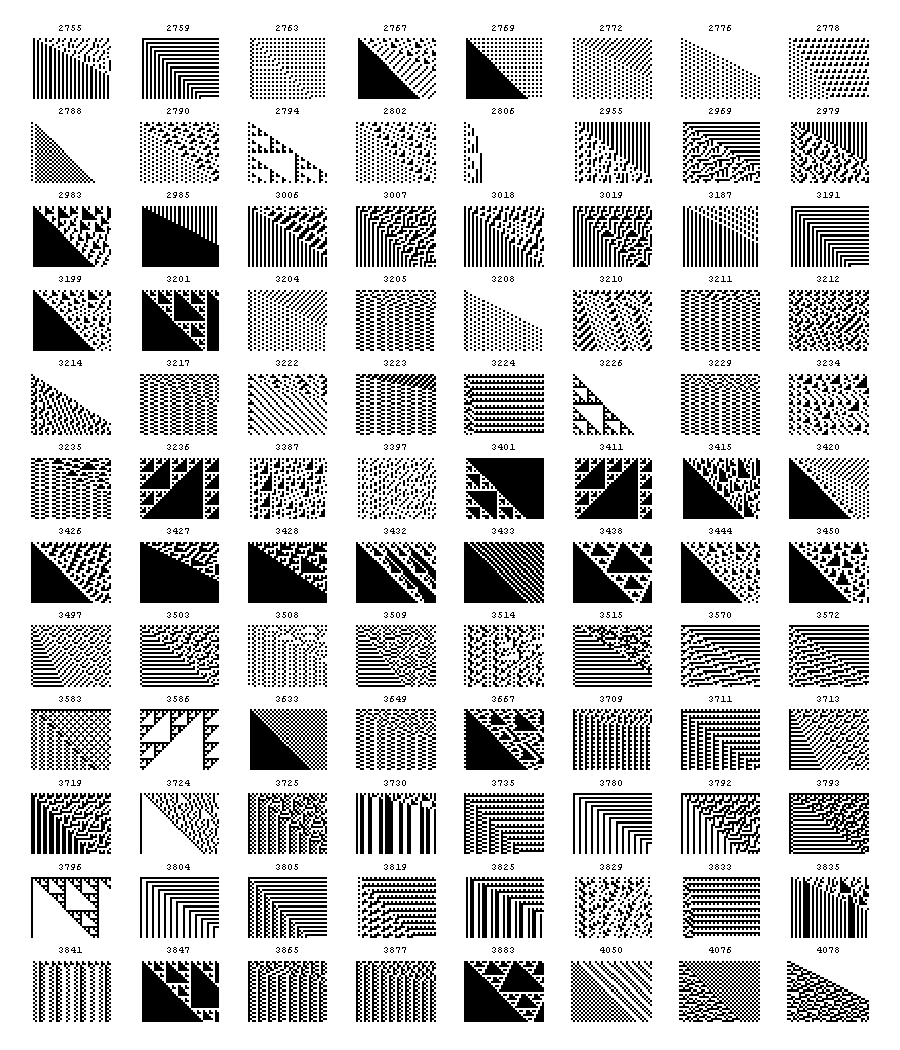Of these, there are then many examples of nesting—often quite elaborate and elegant (e.g. 27601).

But then there are also lots of rules that show much more complex behavior. I attach a few examples. Many look extremely random. Several look somewhat like the powers of 3 in base 2 (see page 120), and indeed exactly this rule should occur somewhere among these finite automata. One that piqued my interest was 28126, which looks incredibly like a 45-degree-rotated version of rule 30.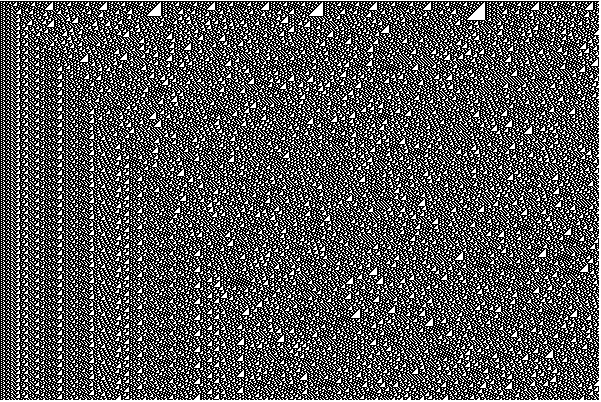There is obviously a lot to investigate here. Different initial conditions for a start. Then an obvious direction is to connect properties of iterated finite automata with properties of underlying finite automata, as studied in traditional automata theory.

Another direction is to think of the finite automata as defining mappings from one symbol sequence to another. Here’s how one can set up such a mapping for symbol sequences of length n:

FAMap[rule_, s0_, n_, k_:2] :=
Table[{i, FromDigits[FAStep[
rule, s0, IntegerDigits[i, k, n]], k]}, {i, 0, k^n - 1}]

And one can set this up as a matrix using:

FAMapMatrix[rule_, s0_, n_, k_:2] :=
Normal[SparseArray[((# + 1) -> 1) & /@
FAMap[rule, s0, n], {k^n, k^n}]]

One can plot such mappings, much like the cellular automaton mappings of page 869R. But in the finite automaton case, the pictures must always have a nested structure. And in fact it is known that they can always be created by 2D substitution systems—presumably using something like the analogy between 1D substitution systems and finite automata on page 891R.

But what is the connection with group theory?

There is a scheme for associating groups with finite automata that Grigorchuk has used since the early 1980s—and which has allowed him to construct a series of very unexpected examples in group theory and elsewhere. (Grigorchuk says that Victor Glushkov—who was a major wheel in Soviet cybernetics and computer development—mentioned the beginnings of such possibilities in the early 1960s.)

Here’s how the scheme works. The basic idea is to associate initial states in a transducer finite automaton with generators in a group. Then applying a transducer finite automaton—say with FAStep[rule, s0, list]—is like multiplying by the generator associated with s0.

To see more about how this works, consider for example automaton 150, with rule:

{{1, 1} -> {2, 0}, {1, 0} -> {1, 1}, {2, 1} -> {1, 1},
{2, 0} -> {2, 0}}

Now label the states a and b rather than 1 and 2, giving:

{{a, 1} -> {b, 0}, {a, 0} -> {a, 1}, {b, 1} -> {a, 1},
{b, 0} -> {b, 0}}

Our evolution above was achieved by starting from the same initial finite automaton state at each step—in effect doing an {a, a, a, a, a, …} evolution. But Grigorchuk’s scheme is to allow an arbitrary sequence of such initial states on successive steps—say {a, b, b, b, a, a, …}. It then turns out that any such sequence can be thought of as corresponding to a word in a group.

For any transducer finite automaton it is fairly clear that such sequences must correspond to words in a semigroup. They correspond to words in a group if the finite automaton is invertible, which can be determined by Det[FAMapMatrix[rule, 1, s]]!=0. With s=2, k=2, 80 of the 256 automata have this property. With s=3, k=2, 9504 out of 46656 have the property.

Now the question is what relations exist in the groups defined by these automata. A relation might say for example that {a, a, a} is equivalent to the identity, which would mean that our evolutions above would have to be periodic with period 3. Or it might say something much less trivial. Typically it does not seem easy to find these relations. In principle it should be possible to find them by a successive approximation in which one looks at symbol sequences of successively larger length n, and sees what relations exist in each case. I didn’t write a program to do this, so I’m not sure how well it might or might not work. But so far as I understand it, the word problem (see e.g. page 1141) for these groups is always solvable, so I believe it follows that such a procedure must eventually converge.

Grigorchuk and collaborators have shown that the 80 invertible automata with s=2, k=2 define a total of 6 distinct groups. Automaton 150 is one that gives the most complicated group: the so-called lamplighter group.

Various other finite automata that give interesting groups are known. In the early 1980s, Grigorchuk used the group defined by s=5, k=2 automaton 8950703898 to show that there are groups for which the number of distinct words of length n grows at a rate intermediate between polynomial and exponential. As I understand it, there are now s=2, k=3 examples known with this property.

There is considerably more to this story—only a small part of which I know. But for right now it seems interesting just to study iterated finite automata in the same kind of way that I studied cellular automata.

And for purposes of group theory it may perhaps be of interest to look at the simplest iterated finite automata that yield complex behavior—since these may correspond to the simplest groups with various kinds of unexpected properties. And indeed in the spirit of PCE one might expect that as soon as s > 2 or k > 2 there would be examples of—in some sense—groups with all interesting properties.